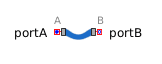Unit Delay - MapleSim Help

Unit Delay

Unit Delay BlockDescription The Unit Delay component implements a unit delay; the output signal $y$ is the input signal $u$ of the previous sample instant. Before the second sample instant, each output signal is equal to the initial value defined by the vector parameter ${y}_{0}$. The Signal Size parameter assigns the dimension of the input and output connectors.Connections

 Name Description Modelica ID $u$ Real input vector u $y$ Real output vector yParameters

 Name Default Units Description Modelica ID Sample Period $0.1$ $s$ Sample period of component samplePeriod Signal Size $1$ Dimension of input and output signals signalSize ${T}_{0}$ $0$ $s$ First sample time instant startTime ${y}_{0}$ $\mathrm{zeros}\left(\right)$ Vector of initial values for each output signal y_startModelica Standard Library The component described in this topic is from the Modelica Standard Library. To view the original documentation, which includes author and copyright information, click here.